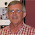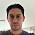## Wednesday, May 30, 2012

### More About Spurious Regressions

Students of econometrics are familiar with the "spurious regression" problem that can arise with (non-stationary) time-series data.

As was pointed out by Granger and Newbold (1974), the “levels” of many economic time-series are integrated (or nearly so), and if these data are used in a regression model then a high value for the coefficient of determination (R2) is likely to arise, even when the series are actually independent of each other.

They also demonstrated that the associated regression residuals are likely to be positively autocorrelated, resulting a very low value for the Durbin-Watson (DW) statistic. There was a time when we tended to describe a “spurious regression” as one in which R2 > DW.

Eventually, Phillips (1986) came up with an elegant formal analytical explanation for the behaviours of the OLS regression coefficient estimator, the usual t-statistics and F-statistic, and the R2 and DW statistics in models estimated using non-stationary time-series data.

Specifically, Phillips introduced a new asymptotic theory that he then used to prove that in a spurious regression the DW statistic converges in probability to zero, the OLS parameter estimators and R2 converge to non-standard limiting distributions, and the t-ratios and F-statistic diverge in distribution, as T ↑ ∞ .

So, effectively Phillips “solved” the spurious regression problem. Moreover, he proved that we can't avoid the adverse consequences of modelling with integrated (but not cointegrated) data simply by increasing our sample size.

We now know that if the data are integrated, but not cointegrated, then they have to be filtered (typically, differenced) before being used in a regression model.

On the other hand, if the data are cointegrated, then we can legitimately estimate a model using the levels of the data. This will be the long-run equilibrating relationship between the variables. Alternatively, we can difference the data but include an "error correction term" in the regression model. The error correction model captures the short-run dynamics of the relationship between the variables.

Perhaps not surprisingly, various other statistics that we routinely report as part of our regression results also have strange properties when the data are integrated. That's to say, if we inadvertently estimate a "spurious regression", there'll be some other warning signals.

These are less well-known than the R2, DW, t and F results noted above. For example, in Giles (2007) I proved the following results:
• The Jarque-Bera (JB) normality test. When applied to a "spurious regression" model, (T−1JB) converges weakly as, T ↑ ∞ and so JB itself diverges at the rate “T”.
• The Breusch-Pagan-Godfrey homoskedasticity test. When applied to a "spurious regression" model, the statistic for the TR2 version of the test diverges  at the rate "T" as T ↑ ∞ . The same is true for the (SSR/2) version of the test.

So, testing for the normality or homoskedasticity of the errors in a "spurious regression" will always lead to a rejection of the associated null hypotheses, for large enough T, whether these hypotheses are false or true.

Again, notice that these unfortunate properties are still there even if the sample size is infinitely large. They're driven by the characteristics of the data, not the amount of data that's available.

Of course, one could argue that there's really no excuse for estimating "spurious regressions" these days. We have a whole raft of tests for non-stationarity and cointegration. However, many of these tests have quite low power, and they often lack robustness when there are structural breaks in the data, for example. So, "spurious regressions" can still occur, and the more warning signals we have, the better.

References

Giles, D. E. A., 2007. Spurious regressions with time-series data: Further asymptotic results. Communications in Statistics - Theory and Methods, 36, 967-979. (Free download here.)

Granger, C. W. J. and P. Newbold, 1974. Spurious regressions in econometrics. Journal of Econometrics, 2, 111-120.

Phillips, P. C. B., 1986. Understanding spurious regressions in econometrics. Journal of Econometrics, 33, 311-340.

© 2012, David E. Giles

1.spell check..
"Specifically, Phillips introduced a new asymptotic theory that he then used it to prove"

1.Thanks - fixed!

2.I don't know if you are open to suggestions about topics to cover; if you are, how about problems and implications of unit roots and cointegration with TSCS data? I've often seen reference to Holtz-Eakin, Newey & Rosen (1988: "Estimating vector autoregressions with panel data", Econometrica, 56) as a justification for ignoring unit roots in this context. The argument, as I understand it, is that you can rely on asymptotics with regard to the size of the cross-section to take care of consistency even in the presence of unit roots. My gut feeling, and it is no more than that, is that this amounts to a hand-waving dismissal of some serious problems for estimation and inference.

1.Marcel: Always open to suggestions. I'll see what I can do!

3.The headlined subject of this post is of great interest to me -- a non-specialist. But this communication suffers greatly from the absence of a single real-world example of, e.g. "integrated" or "co-integrated" data, "differencing" (?), "error-correction model," etc. etc.

I'm not trying to be querulous. It's just that not all your interested readers are specialists. And the extra intellectual effort required to provide examples would help us...

1.Thanks for the comment. O.K. - brief post coming up later today with definitions and an example for you. :-)

4.This comment will not be robust to the criticism of the previous commenter.

I've never understood why it's okay to estimate a VAR in levels when a Johansen test gives evidence of (strictly) between 0 and N cointegrated relationships. Sure, you're implicitly estimating the cointegrating vector, which would be an omitted variable if you'd estimated in differences. But what about all the relationships that aren't cointegrated? Aren't you inviting spurious regressions in those?

1.Fibby: Interesting comment. I don't have an immediate answer. Suggestions, anyone?

5.Dear Dave,

Thank you for this usefull post. Perhaps you could spin your conclusion and add the case of those doing spurious regressions wittingly.
I saw in some papers that the dependent is I(0), even accounting for structural breaks, and the independents are I(1). I wonder if their results have a mining when they regress all the variabes in level. One of the justification they provide is that they control for autocorrelation by adding an AR term and then they test the residuals for stationnarity. IS it a correct way to do? Thanks.

1.Thanks for the comment. No, it's certainly NOT correct.

If the dependent variable is I(0) and the regressors are I(1), then the regression will be "unbalanced" (and the results will be meaningless) unless the regressors happen to be cointegrated.

2.sorry, a follow up question. if the regressors cointegrated with different coefficients, will the original regression still meaningful? thanks

3.If the data are cointegrated, the regression will be meaningful, and in fact OLS will be "super-consistent".

6.can u please define spurious regression in time series econometrics ?

7.It's a regression in which the dependent variable and the regressors are non-stationary, but NOT cointegrated.

8.Hi Prof. Giles

You have mentioned that 'if the data are integrated, but not cointegrated, then they have to be filtered (typically, differenced) before being used in a regression model'

I would like to know whether this is applicable if I am using a TY procedure for Granger causality testing using VAR.

1.No - that's the whole point of the TY procedure. With their modification to the VAR you MUST estimate it in the levels, whether the series are integrated or nor, even if they're not cointegrated.

2.Hi Prof. Giles

Thanks for your kind reply. Could you throw some light on forecasting using non stationary VAR model

9.Dear Prof. Gelis
Thank you for this very useful post
I would like to ask that if I find R2 > DW, I can directly decide it is a “spurious regression”. I mean it is almost enough.
Thank you again

1.No, you can't.

10.Dear Prof. Giles,

Can I use one of the tests mentioned in your article to test for spurious regression and conclude that variables are cointegrated if there is no spurious regression?

Best regards,
Pravin

1.No - just use a conventional cointegration test.

11.Dear Prof. Giles

How can change your post if considered the paper of Sims, Stock and Watson of 1990 over the level estimation in time series? There they include VAR example only. In an univariate model it is possiblity?

Thanks,

Paúl Carrillo

1.The post applies to both situations.